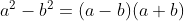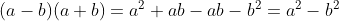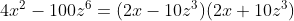## Difference of two squares

Source: Wikipedia, the free encyclopedia

The difference of two squares is a squared number subtracted from another squared number. Every difference of squares may be factored according to the identityThe above identity can be easily proven, sinceThis identity is one of the most commonly used in mathematics and the variables a and b can be replaced by any expression to factor more complex binomials:## Repeated use of factoring by the difference of two squares:

The identity can be applied more than once if the resulting expressions have the form of a difference of two squares:Here the second binomial was factored since it's a difference of two squares